# The HPGENSELECT Procedure

### The LASSO Method of Model Selection

Subsections:

#### LASSO Selection

The HPGENSELECT procedure implements the group LASSO method, which is described in the section Group LASSO Selection. This section provides some background about the LASSO method that you need in order to understand the group LASSO method.

LASSO (least absolute shrinkage and selection operator) selection arises from a constrained form of ordinary least squares regression in which the sum of the absolute values of the regression coefficients is constrained to be smaller than a specified parameter. More precisely, let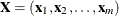denote the matrix of covariates, and let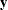denote the response. Then for a given parameter t, the LASSO regression coefficients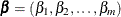are the solution to the constrained least squares problem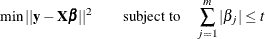For generalized linear models, the LASSO regression coefficientsare the solution to the constrained optimization problem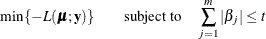where L is the log-likelihood function defined in the section Log-Likelihood Functions.

Provided that the LASSO parameter t is small enough, some of the regression coefficients will be exactly zero. Hence, you can view the LASSO method as selecting a subset of the regression coefficients for each LASSO parameter. By increasing the LASSO parameter in discrete steps, you obtain a sequence of regression coefficients for which the nonzero coefficients at each step correspond to selected parameters. For more information about the LASSO method, see, for example, Hastie, Tibshirani, and Friedman (2009).

#### Group LASSO Selection

The group LASSO method, proposed by Yuan and Lin (2006), is a variant of LASSO that is specifically designed for models defined in terms of effects that have multiple degrees of freedom, such as the main effects of CLASS variables, and interactions between CLASS variables. If all effects in the model are continuous, then the group LASSO method is the same as the LASSO method.

Recall that LASSO selection depends on solving a constrained optimization problem of the formIn this formulation, individual parameters can be included or excluded from the model independently, subject only to the overall constraint. In contrast, the group LASSO method uses a constraint that forces all parameters corresponding to the same effect to be included or excluded simultaneously. For a model that has k effects, let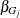be the group of linear coefficients that correspond to effect j in the model. Then group LASSO depends on solving a constrained optimization problem of the form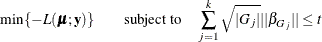where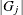is the number of parameters that correspond to effect j, and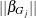denotes the Euclidean norm of the parameters,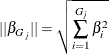That is, instead of constraining the sum of the absolute value of individual parameters, group LASSO constrains the Euclidean norm of groups of parameters, where groups are defined by effects.

You can write the group LASSO method in the equivalent Lagrangian form, which is an example of a penalized log-likelihood function: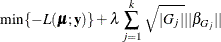The weight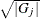was suggested by Yuan and Lin (2006) in order to take the size of the group into consideration in group LASSO.

Unlike LASSO for linear models, group LASSO does not allow a piecewise linear constant solution path as generated by a LAR algorithm. Instead, the method proposed by Nesterov (2013) is adopted to solve the Lagrangian form of the group LASSO problem that corresponds to a prespecified regularization parameter
. Nesterov’s method is known to have an optimal convergence rate for first-order black box optimization. Because the optimalis usually unknown, a series of regularization parameters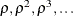is employed, where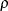is a positive value less than 1. You can specifyby using the LASSORHO= option in the PROC HPGENSELECT statement; the default value is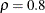. In the ith step of group LASSO selection, the value that is used foris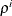.

A unique feature of the group LASSO method is that it does not necessarily add or remove precisely one effect at each step of the process. This is different from the forward, stepwise, and backward selection methods.

As with the other selection methods that PROC HPGENSELECT supports, you can specify a criterion to choose among the models at each step of the group LASSO algorithm by using the CHOOSE= option in the SELECTION statement. You can also specify a stopping criterion by using the STOP= option in the SELECTION statement. If you do not specify either the CHOOSE= or STOP= option, the model at the last LASSO step is chosen as the selected model, and parameter estimates are reported for this model. If you request an output data set by using an OUTPUT statement, these parameter estimates are used to compute predicted values in the output data set.

For more information, see the discussion in the section SELECTION Statement in SAS/STAT 14.1 User's Guide: High-Performance Procedures.

The model degrees of freedom that PROC HPGENSELECT uses at any step of the LASSO are simply the number of nonzero regression coefficients in the model at that step. Efron et al. (2004) cite empirical evidence for doing this but do not give any mathematical justification for this choice.

Some distributions involve a dispersion parameter (the parameterin the expressions for the log likelihood), and in the case of the Tweedie distribution, a power parameter. These parameters are not estimated by the LASSO optimization algorithm, and are set to either the default value or a value that you specify. You can use the MODEL statement options PHI= to set the dispersion to a fixed value and P= to set the Tweedie power parameter to a fixed value.